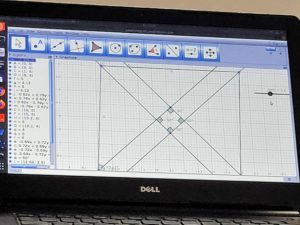## Fraction multiplication V2

From the STEMland scaling session with Swathi on Friday, the input received was to make the multiplication to be a perfect square to show that 1/4 is smaller than 1/3.

Murali from 9th grade implemented the change:

## Scratch Madala at Aikiyam school

Pratap

This week me and Murali went to Aikiyam to teach shapes using scratch. We started with the drawing a straight line. Then asked children to draw a triangle . Children took some time and then figured out the angle to draw a triangle. Then we asked them to draw the following shapes like square, pentagon, hexagon, heptagon , octagon. Then using this shapes we asked then to draw mandala. Children were interested to  draw their own mandalas . Children understood the concept of repeat loop. They were familiar with the  pen function in the scratch. Children were able to connect the angles for different shapes.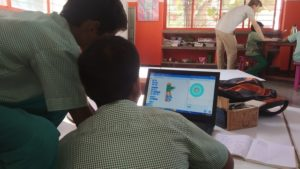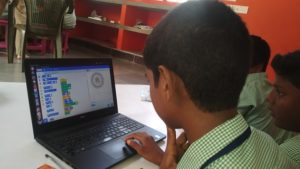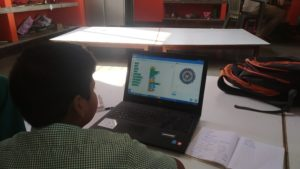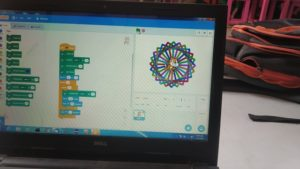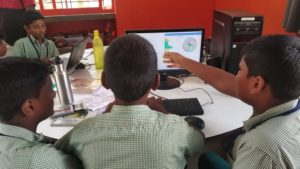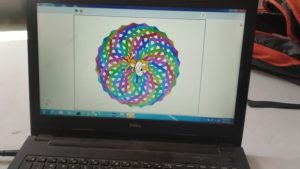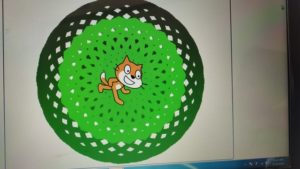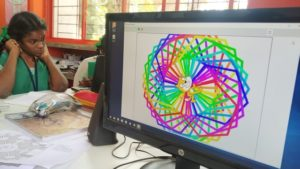## STEM land meeting with Swati Sircar

~Poovizhi, Murali and Arun

This week it was a large group of people in the meeting. Coordinators from Aikiyam, Udavi, Isai ambalam and AITI joined the meeting. Swati Sircar joined the meeting online. At first Sundar demonstrated Scratch projects on finding LCM created by 8th grade and program on Graph created by 9th grade children which asks the user to find the point and completes by drawing a bear. Then we listed few topics to discuss with Swati. Swati explained about Trignometry. She said we can ask children to come up with 6 possible ratios from a right angle triangle and map the names.

Then different methods of teaching multiplications

7×9 = 7×10-7

To explain and use multiplication in

1. Scaling
3. Cartesian product (Choices of combinations)
4. Area (rows and column)
5. Rate (price and qty)

Then we moved to Division and she explained how to spit 11/3. For example to draw three circles and draw apples in each circle equally and the remaining is the reminder.

Then we discussed about simplification. For simplification children need to learn divisibility rules for 2,3,4,5,9,10 and 11

Followed with we had a conversation on negative exponential

2^-x = 1/2^x

(1/2)^-5 = (2)^5

2^3 = 8

2^2 = 4

2^1 = 2

2^0 = 1

2^-1 = 1/2

2^-2 = 1/4

2^-3 = 1/8

## STEM land meeting with all STEM coordinators

~Murali and Poovizhi

STEM land meeting with all STEM coordinators

This  Friday (29/11/2019) we had an interesting sharing session. Abilash, Ranjith and Sivaraman presented an experiment on Electrolysis through which they generated hydrogen from water using salt and electricity. It was new for us and we learnt how oxygen and hydrogen splits from water.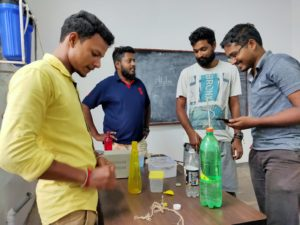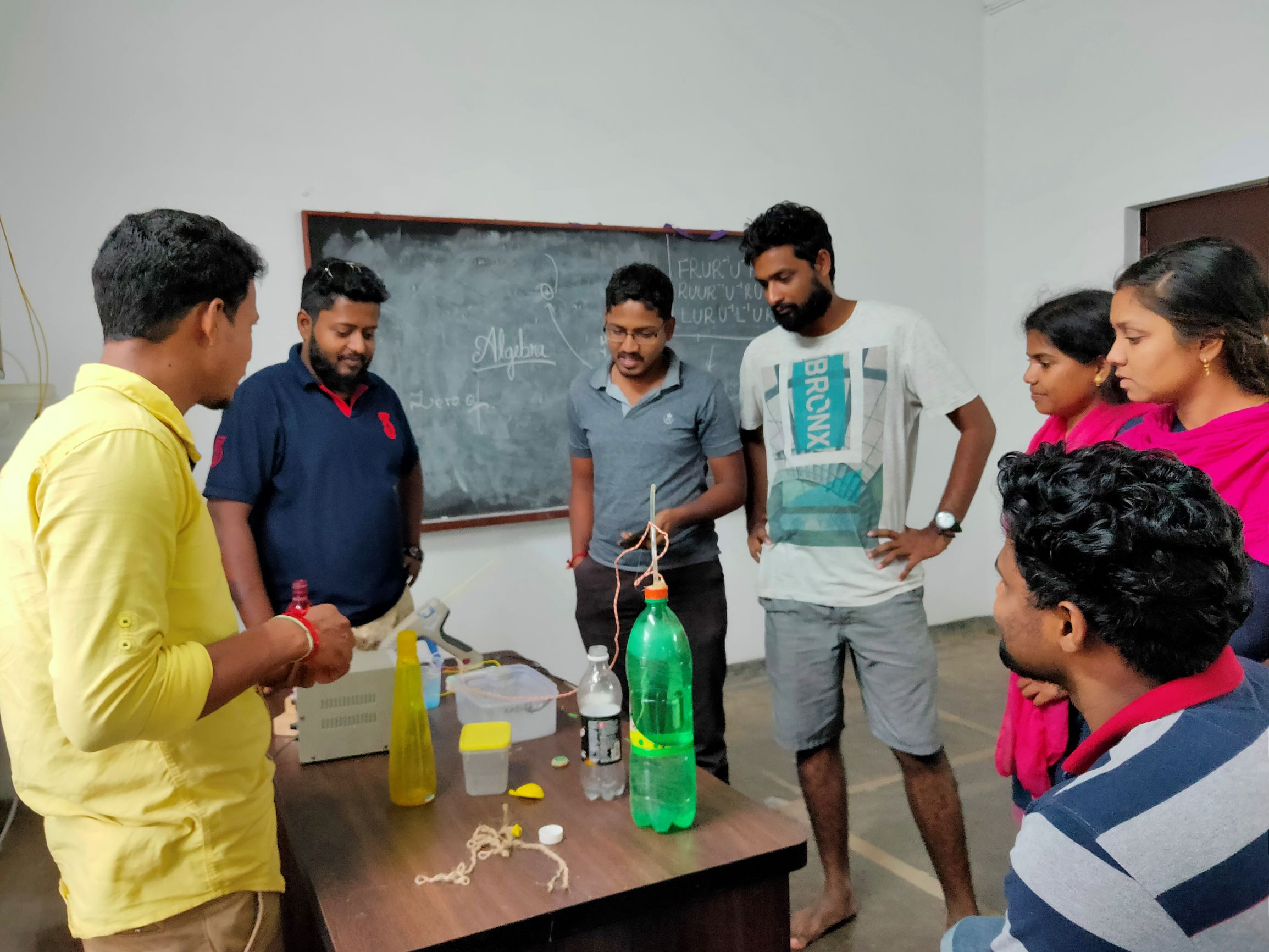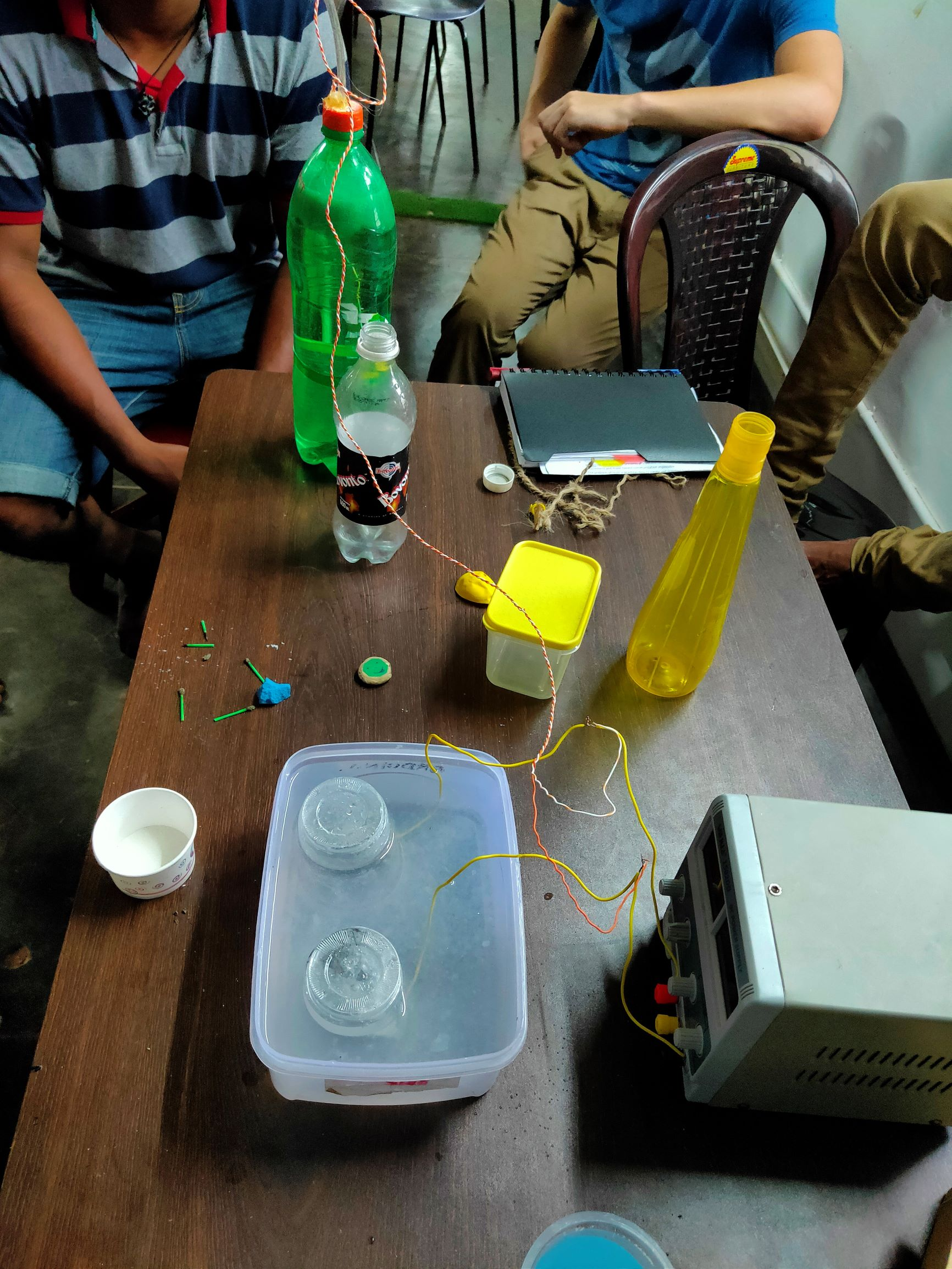After this practical experiment, Sundar from Udavi Stemland presented a scratch project on a graph in which the sprite moves to a particular point and asks the user to enter the (x,y) coordinates. Once the user enters the correct coordinates, it moves to the next point and so on. If the user completes until the end it draws an image.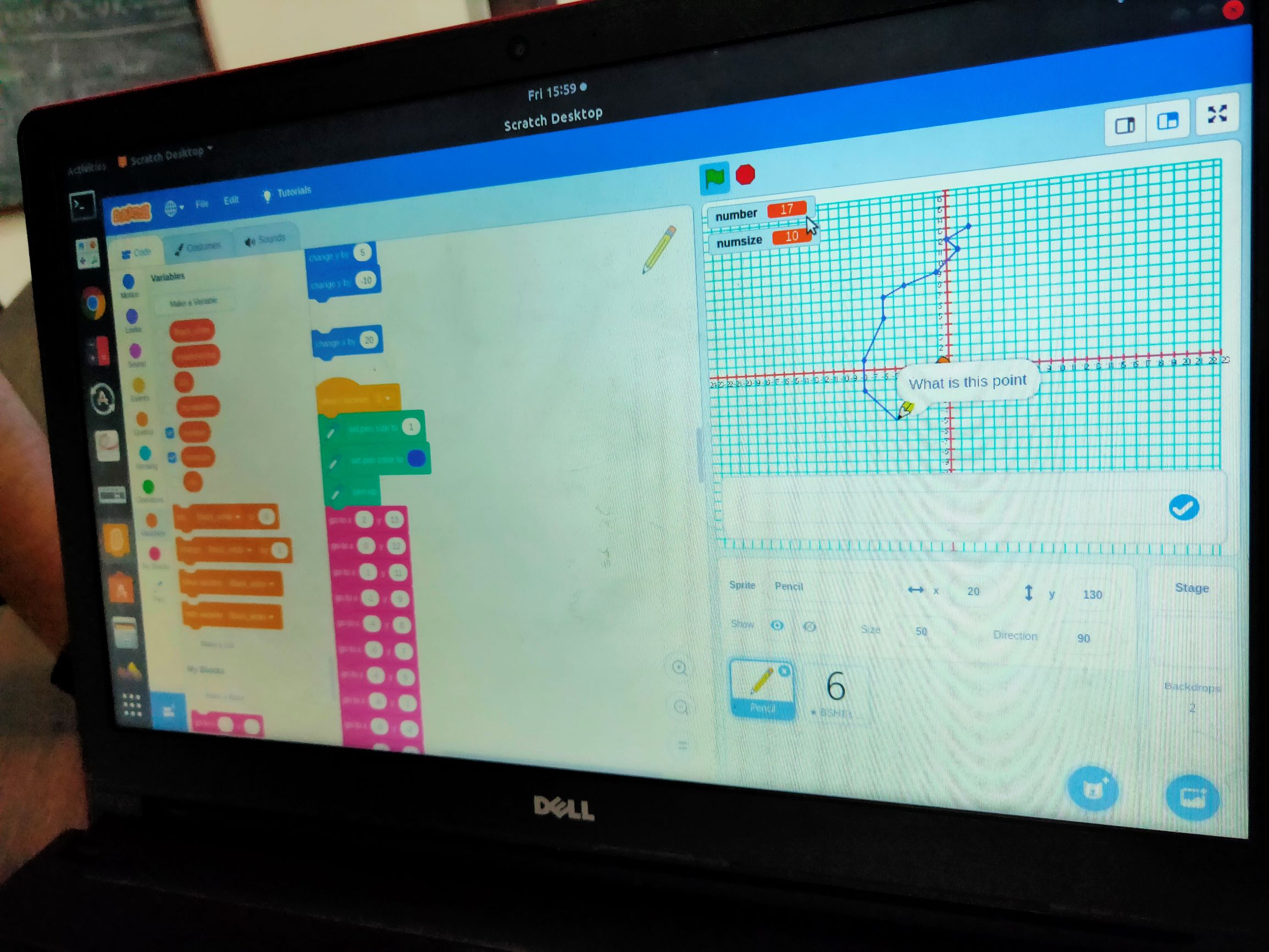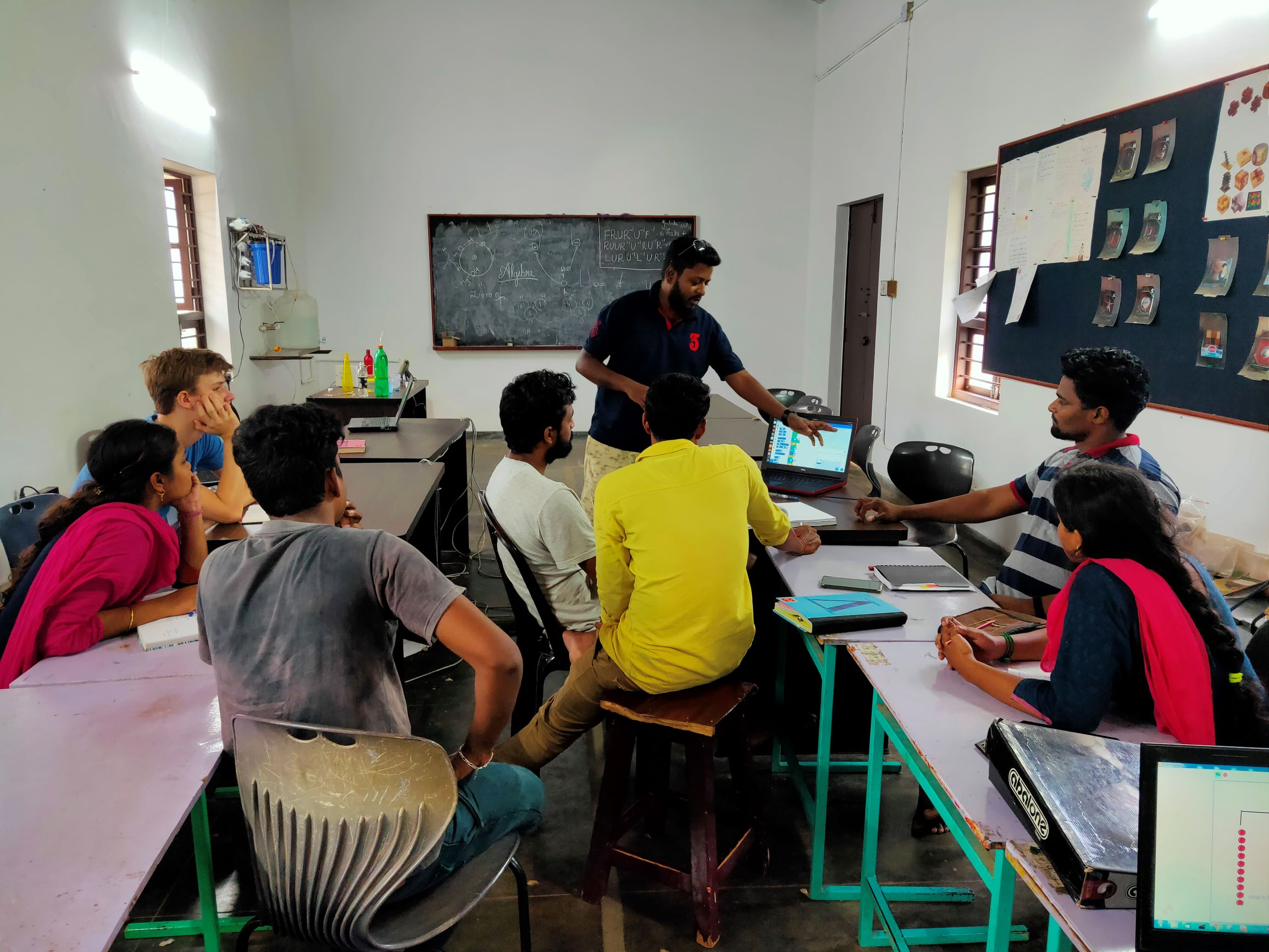Following this, we had another scratch project on algebraic expression. It is a game where the user is supposed to find the value of the given objects on both sides of a weighing scale.

## STEM land meeting with all STEM coordinators

~ Murali and Poovizhi

Sundar (Udavi school STEM land coordinator) gave a demonstration on learning Algebra using weighing scale. He used few sketch pens and weighing cubes (1gm) . For example one side had 10 grams and the other side had 5 sketch pens when both were weighing equal. He asked us to find the weight of each sketch pen. Then we discussed and solved few such examples in solveme and came up with all possible algebraic equations.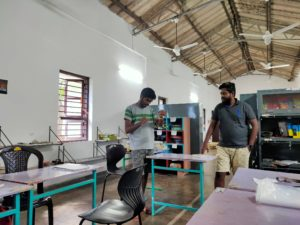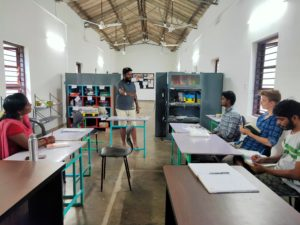Saravanan from Aikiyam school had brought a star tetrahedron in which children had learnt about pyramids and triangles.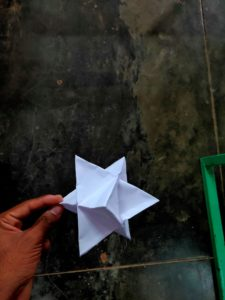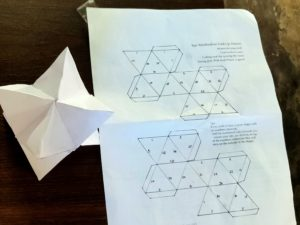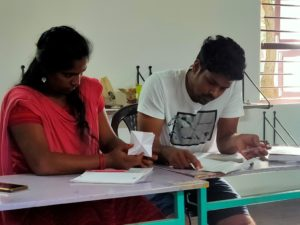Arun who is coordinating with AIIT explained about parallelogram and showed how a rectangle can be formed by bisecting the angles of the parallelogram.